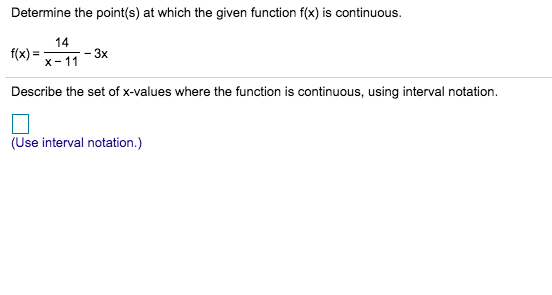# Determine the point(s) at which the given function f(x) is continuous.14-3xX 11f(x)Describe the set of x-values where the function is continuous, using interval notation.(Use interval notation.)

Question
21 views

Can i get help with this problem step by step?help_outlineImage TranscriptioncloseDetermine the point(s) at which the given function f(x) is continuous. 14 -3x X 11 f(x) Describe the set of x-values where the function is continuous, using interval notation. (Use interval notation.) fullscreen
check_circle

Step 1

Refer to the question,

To determine the points where the provided function is continuous .

Step 2

Here to check the continuity of the function at a point the left and limit = right hand limit = value of function at that point that is,

Step 3

As the given function is a polynomial function so it define for all value of x ad will be continuous at each ...

### Want to see the full answer?

See Solution

#### Want to see this answer and more?

Solutions are written by subject experts who are available 24/7. Questions are typically answered within 1 hour.*

See Solution
*Response times may vary by subject and question.
Tagged in

### Continuity